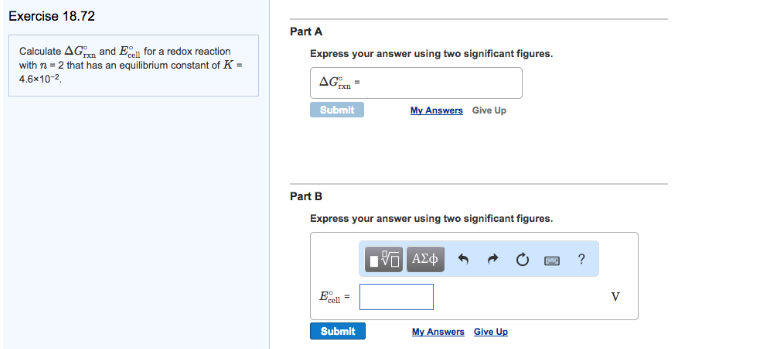# Problem: Calculate ΔG°rxn and E°cell for a redox reaction with n = 2 that has an equilibrium constant of K = 4.6 x 10-2. Express your answer using two significant figures.

###### FREE Expert Solution
99% (34 ratings)###### Problem Details

Calculate ΔG°rxn and E°cell for a redox reaction with n = 2 that has an equilibrium constant of K = 4.6 x 10-2